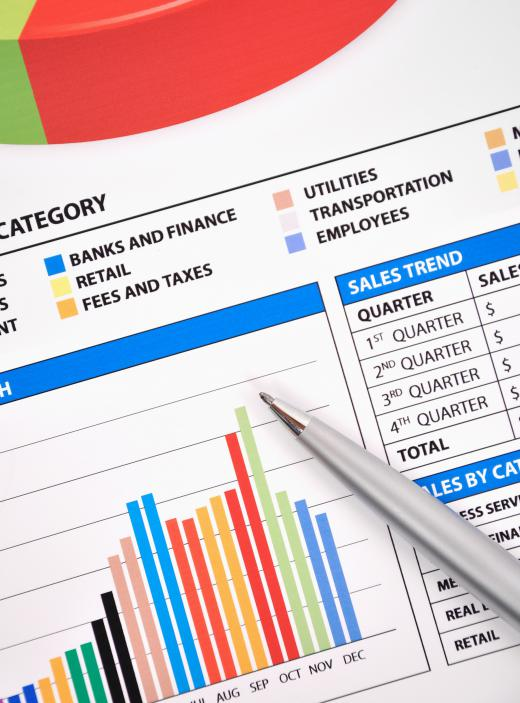Finance
Fact Checked

# What is Gross Margin?

Jessica Reed
Jessica Reed

Gross margin, also known as gross profit margin, shows a company how much money they made after the cost of manufacturing and selling their products is subtracted. The basic formula involves taking the amount of money a company made total over a period of time, such as a year, and then finding out how much money was spent producing and selling each item sold that year. Subtract the cost from the money earned and the company has a rough estimate of how much money they actually made for the year.

Several advantages of calculating gross margin exist for both the company and its investors. Investors want to put their money in a company that has the highest gross margin, meaning it made the most money. The business can also use gross margin to monitor its progress and see what areas need to be controlled. For example, a company may realize the cost of manufacturing a certain project costs more than it's worth. The company can then find a cheaper way to manufacture the product or stop selling the product.Investors want to put their money in a company that has the highest gross margin, meaning it made the most money.

A percentage value can also represent gross margin. This percentage shows the company how much money it is making when compared to how much it has spent. The percentage represents a ratio of money earned to money spent. It's important to keep in mind that costs unrelated to making or selling the product, such as paying employees and renting office space, are not considered in the equation.

To determine the company's gross margin percentage, the cost of all the items sold is subtracted from the company's revenue for those items. Revenue simply means the total amount of money the company made. If the company wants to calculate the gross margin percentage of one specific item, it will look at the money spent and made only on that item.

After subtracting the cost of the items from the revenue made on them, that number is divided by the revenue number. For example, if a company spent \$10,000 US Dollars (USD) and earned \$15,000 USD, it would subtract \$10,000 USD from \$15,000 USD, which equals \$5,000 USD. Then it would divide \$5,000 USD by the original revenue, which was \$15,000 USD. This would give a gross margin of 33%.

Expressing the gross margin in a ratio format simply places the total revenue minus cost of items over the total revenue. In this example, the ratio of gross margin is \$5,000/\$15,000 USD. Reducing this fraction gives a more manageable ratio of 1/3. Thus the company's gross margin is 1/3 or 33%. A negative answer would mean the company was losing money instead of earning it.

## You might also Like